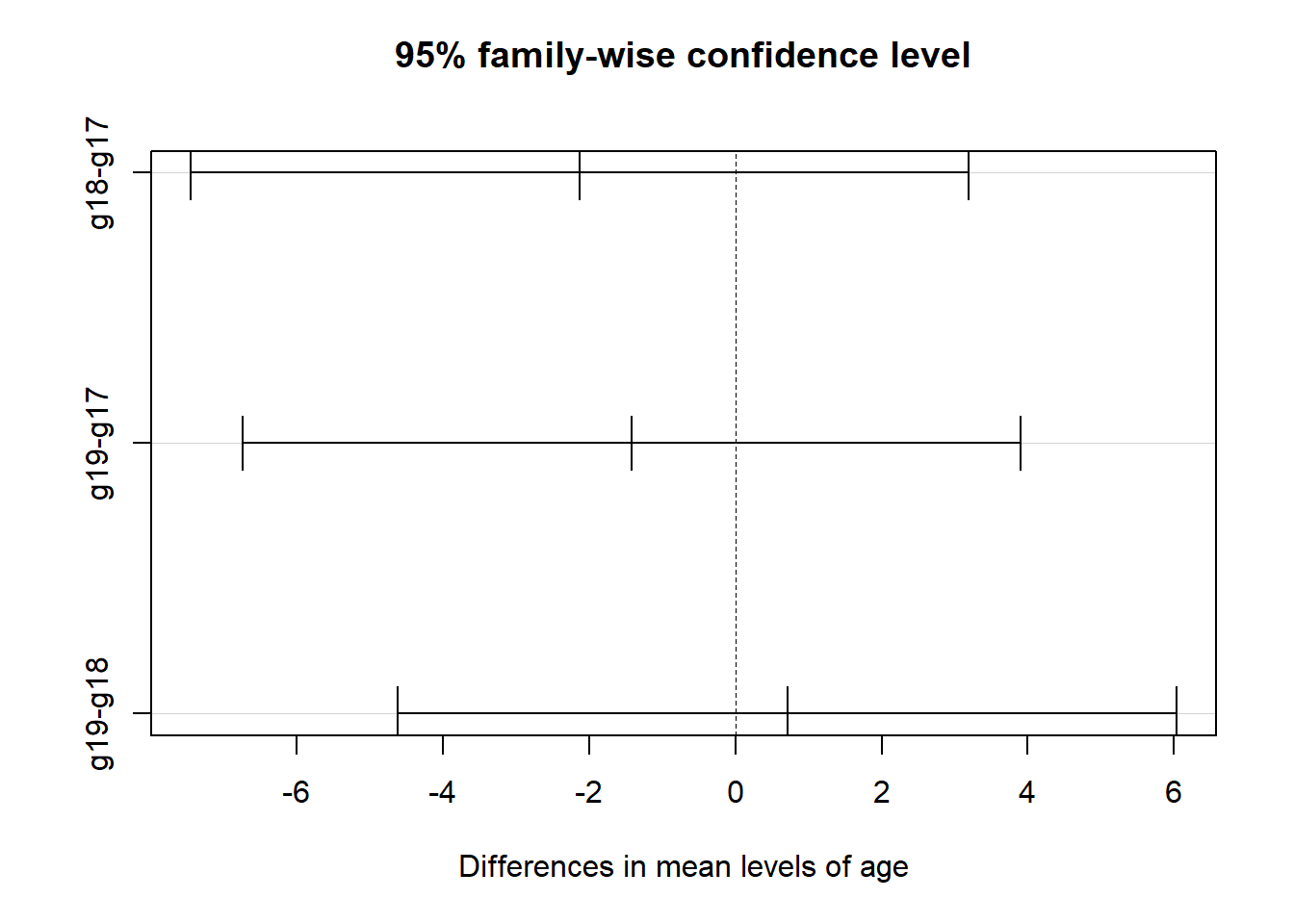# Objective

The purpose of this example is to demonstrate the similarities between one-way ANOVA and linear regression models. After teaching intro stats to undergrads for a decade, I finally saw this magical (and simple, previously invisible to me) connection – and needed to share!

These examples use synthetic data, so we know what the properties of the distributions are, and know what the answers should be even before we start the problem.

## Groups with Different Means

First, let’s simulate some data. I used anthropometry (body measurements) data from the U.S. Centers for Disease Control (CDC), focusing on heights of children in different stages of development. Each row in the height table describes heights (in cm) for a sample (n) of people, reported as the mean for that sample and the standard error of that mean (SEM). I used n and SEM to calculate the sample standard deviations for 2, 7, and 19 year olds, and used this information to generate a synthetic dataset of 20 observations for each group.

library(tidyverse)

# height (cm) from CDC Anthropometry: https://www.cdc.gov/nchs/data/series/sr_11/sr11_252.pdf
set.seed(1234)
hts <- c(91.8,125.4,177.8)
sem <- c(0.38,0.43,0.81)
n <- c(318,193,211)
sigmas <- sem*sqrt(n)

g2 <- rnorm(20,mean=hts,sd=sigmas)
g7 <- rnorm(20,mean=hts,sd=sigmas)
g19 <- rnorm(20,mean=hts,sd=sigmas)

I picked 2, 7, and 19 year olds because 2 year olds are DEFINITELY shorter than 7 year olds, and 7 year olds are DEFINITELY shorter than 19 year olds. A one-way ANOVA to determine whether the average height in any of these groups is different than the others… should say absolutely yes.

The means of the groups are shown below – the 2 years olds are, on average, 90 cm – shorter than the mean of the 7 year olds (122 cm) and much shorter than the mean of the 19 year olds (173 cm). The density plot and comparative boxplot make these observations clear (as if your life experience had not already.) The horizontal line across the boxplots is the mean (132.1) of all n=60 observations.

library(tidyverse)

df <- as_tibble(cbind(g2,g7,g19))
df %>% gather(age,height) %>% ggplot() + geom_density(aes(x=height,fill=age)) +
ggtitle("Heights of 2, 7, and 19 Year Olds")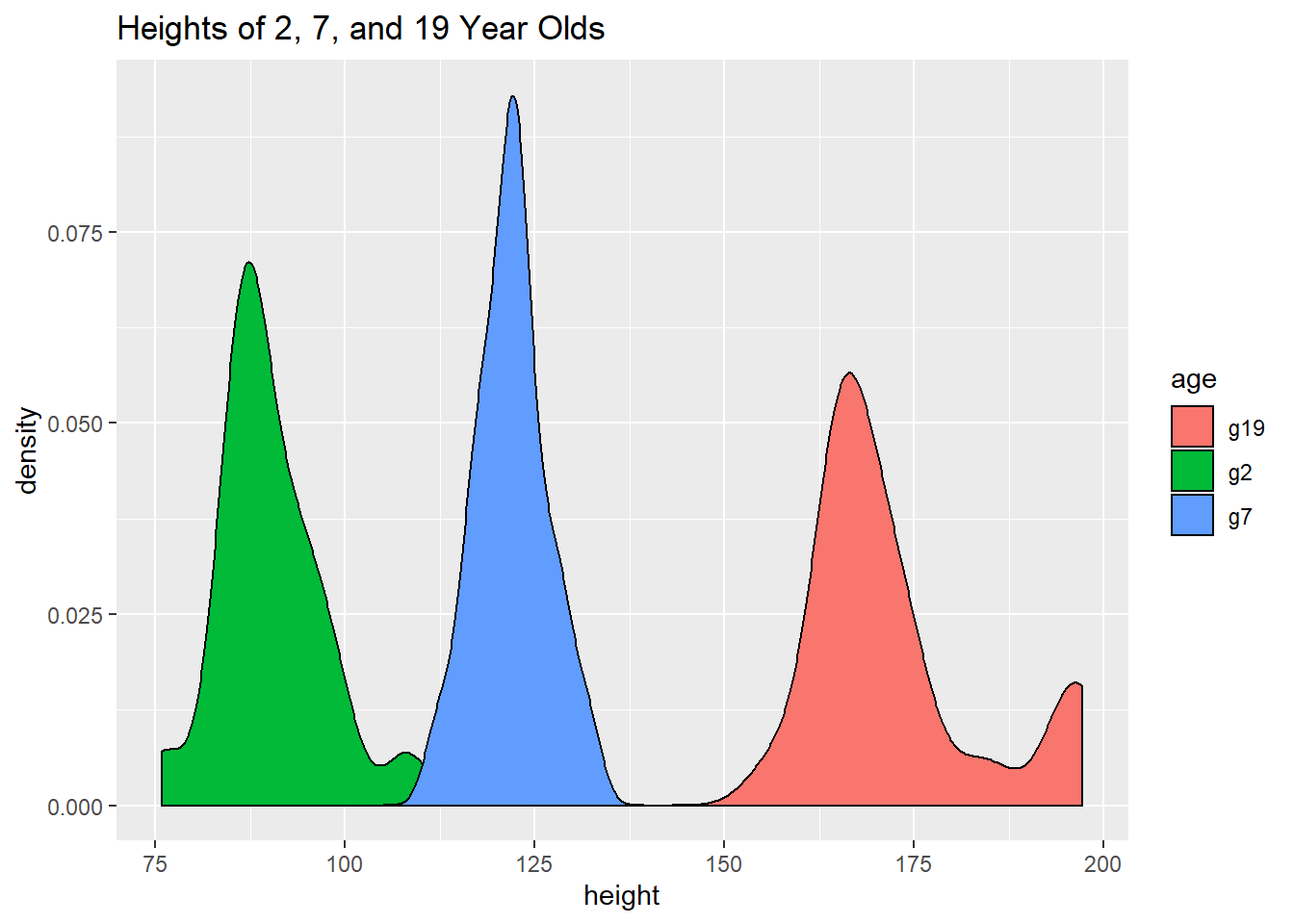df %>% gather(age,height) -> df2
df2 %>% group_by(age) %>% summarize(mean=mean(height))
## # A tibble: 3 x 2
##   age    mean
##   <chr> <dbl>
## 1 g19   173.
## 2 g2     90.1
## 3 g7    122.
df2 %>% ggplot() + geom_boxplot(aes(x=age,y=height)) + geom_hline(yintercept=132.1, lwd=2)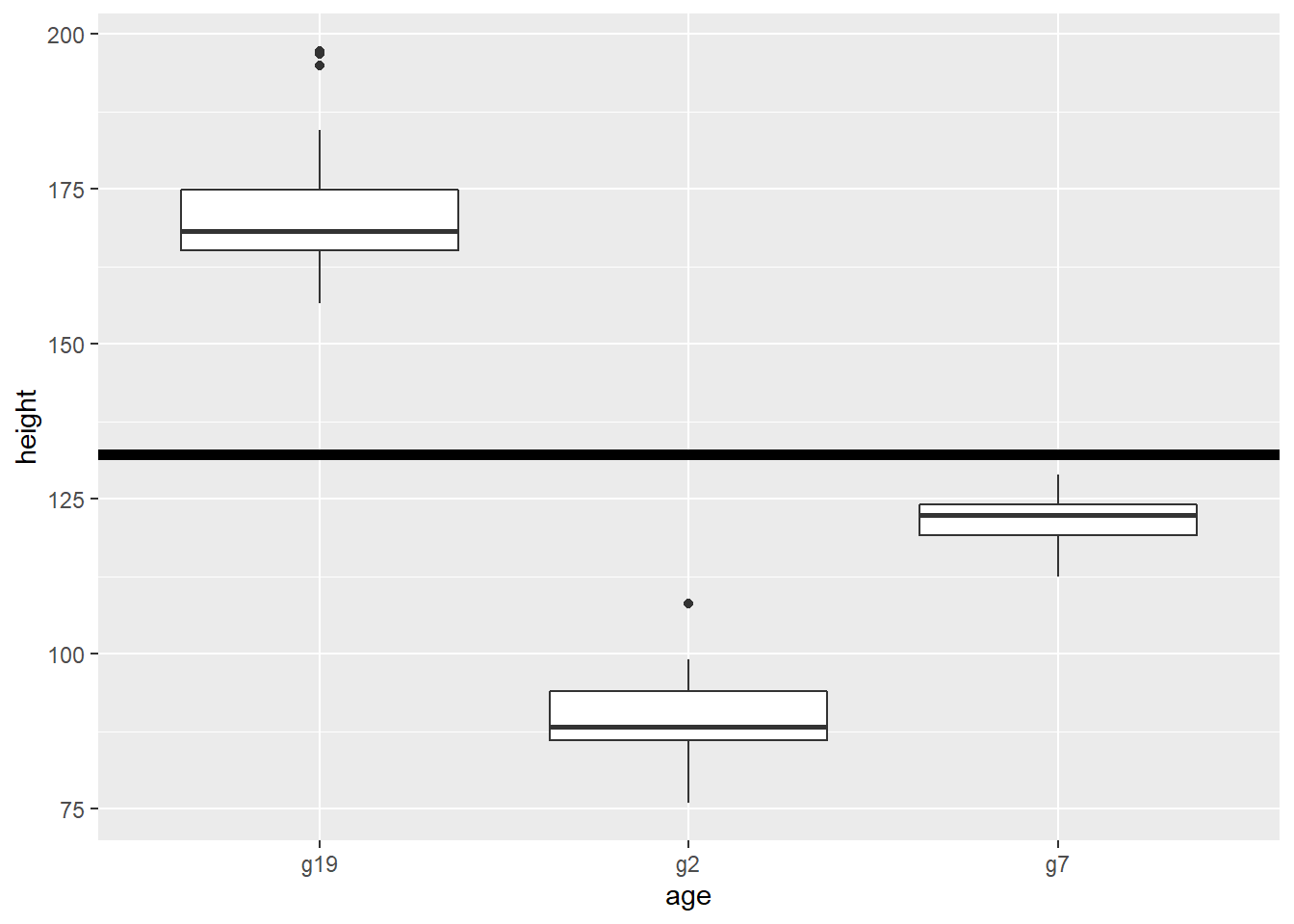### One-Way ANOVA

Since we know the three distributions have very little overlap, we know we’re going to reject the null hypothesis that the mean height in all three groups is the same. (It’s clearly not.) ANOVA gives us the tiny p-value [Pr(>F)] we expect:

summary(aov(height~age, data=df2))
##             Df Sum Sq Mean Sq F value Pr(>F)
## age          2  69188   34594   502.8 <2e-16 ***
## Residuals   57   3922      69
## ---
## Signif. codes:  0 '***' 0.001 '**' 0.01 '*' 0.05 '.' 0.1 ' ' 1

Let’s prove it to ourselves with a Tukey HSD test. If any of the confidence intervals contain zero (cross the dotted line), then the two groups being compared aren’t significantly different. So in this case, we don’t want to see anything cross that vertical dotted line because we expect differences between the groups…

z <- (aov(height~age, data=df2))
TukeyHSD(z)
##   Tukey multiple comparisons of means
##     95% family-wise confidence level
##
## Fit: aov(formula = height ~ age, data = df2)
##
## $age ## diff lwr upr p adj ## g2-g19 -82.47063 -88.78277 -76.15848 0 ## g7-g19 -50.61931 -56.93145 -44.30716 0 ## g7-g2 31.85132 25.53918 38.16346 0 plot(TukeyHSD(z))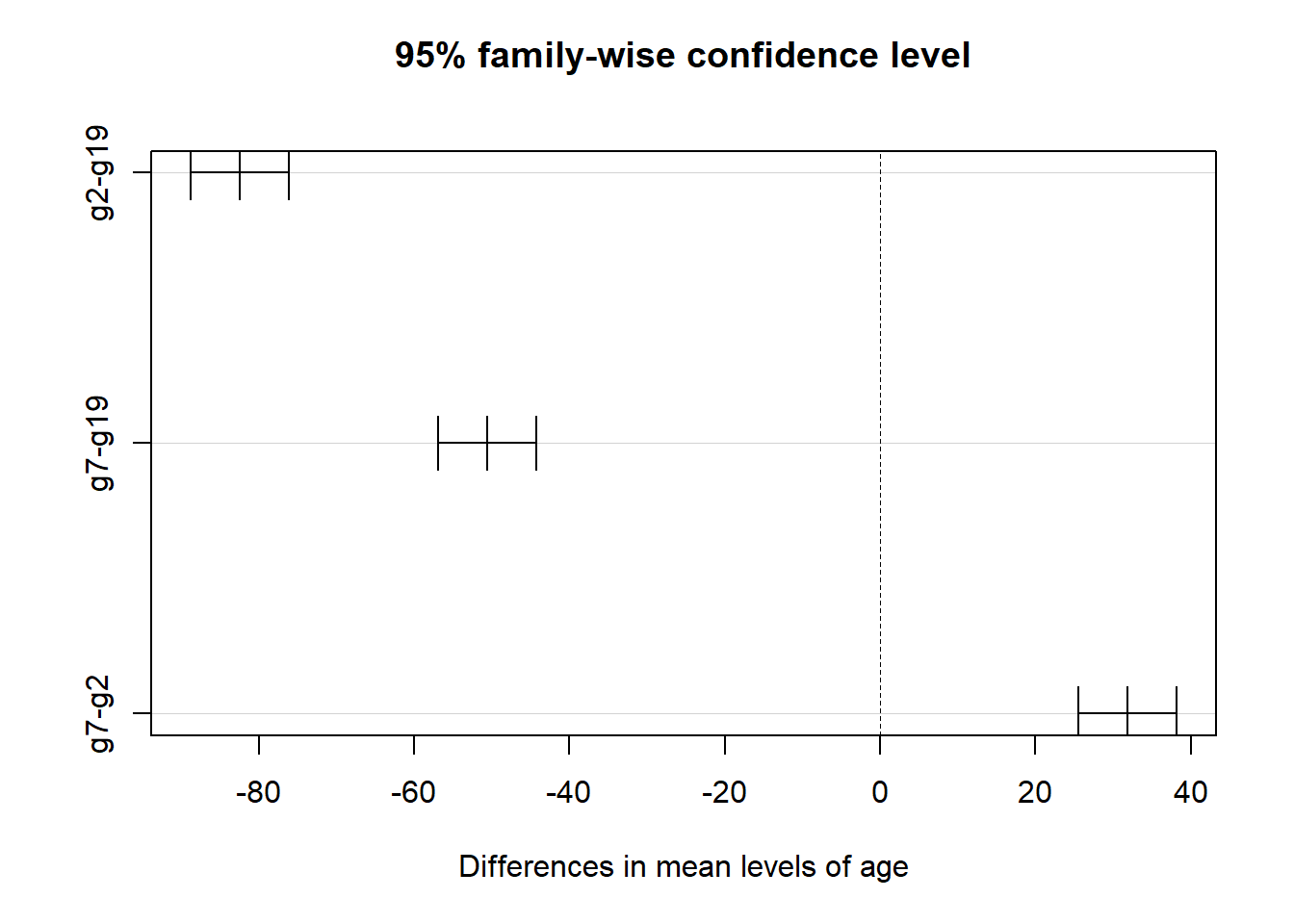… and that’s what happened. ### Why a Linear Model (using lm) Also Works But we can also see whether the groups are different by using a linear model. When you’re doing an inference test to see whether the slope of a best fit line on a scatterplot is significant, the null hypothesis is that the slope of the best fit line is zero (meaning the points are not scattered in a linearly increasing or decreasing pattern.) When your predictor on the x-axis is a categorical variable, it sort of works the same way: just imagine that you’re drawing a best fit line between the y-intercept (or the origin, for a no-intercept model) to the centerpoint of your boxplot, at its median. Does that line have a zero slope? If so, then the means of your groups are not different. Here’s another way to visualize it: connect the dots between the medians of the boxes on your boxplot. If the lines have slopes that are statistically different than zero, at least one of the groups has a mean that’s different than the others (voila; one-way ANOVA). For example, in the boxplot here, the inference test would ask if the slope of the magenta line is different enough from zero to indicate that there is a difference between the group means.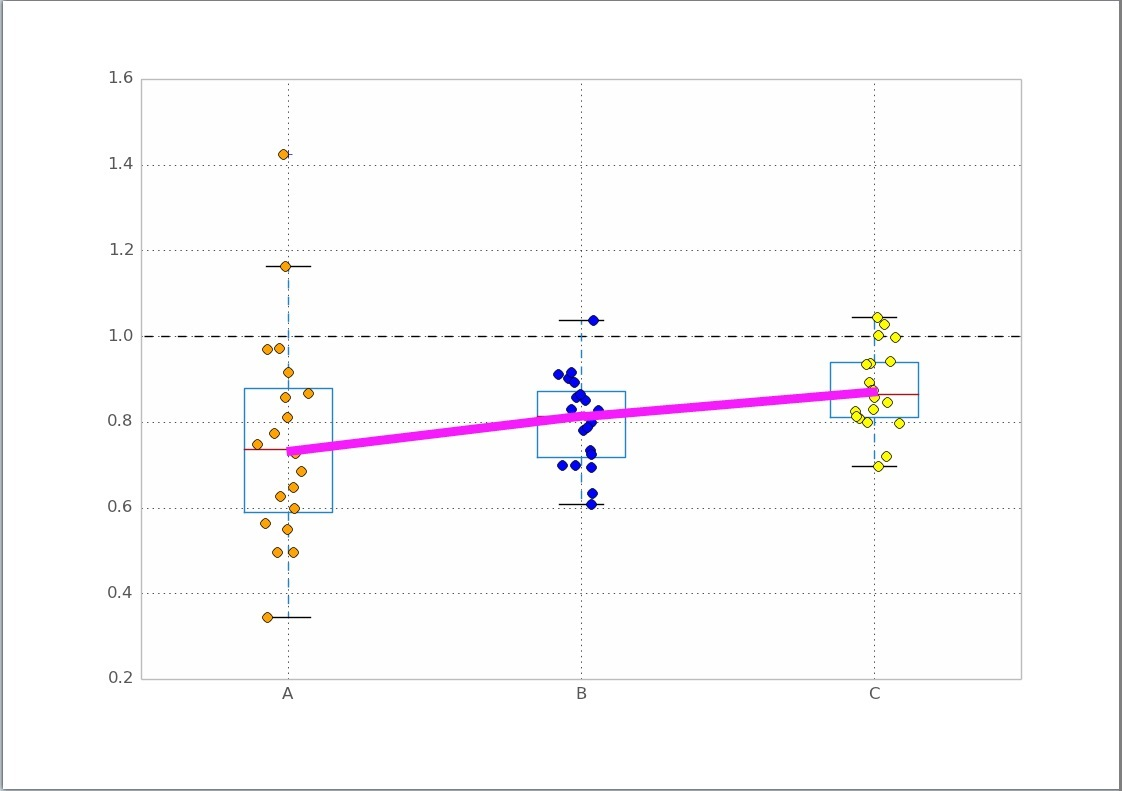### No-Intercept Linear Model In the boxplot, we see a categorical variable on the horizontal (x) axis, and the variable we want to predict, height, on the y axis. We can fit a linear model to the data that will take our group as input, and give us a value for the estimated height for members of that group. For the “no-intercept” model, we set $$\beta_0$$ to 0 in the equation for a linear model: $y = \beta_{0}\: + \beta_{1}{g_1}\: + \beta_{2}{g_2}\: + \beta_{3}{g_3}$ The no-intercept model means that we are pretty sure dependent variable y=0 whenever our independent variables g1, g2, and g3 are all zero. Since the g variables describe membership in a group, if all the g’s are zero, you can’t get a prediction for y. Similarly, if our observation is in group 2 (g2=1), that means g1 and g3 are zero, and the no-intercept equation reduces to: $y = \beta_{2}{g_2}$ We end up with y equal to whatever our estimate for $$\beta_{2}$$ ends up being in the linear model. So let’s create that model and see what it is. fit0 <- lm(height~0+age, data=df2) # no-intercept model; remove 0 for a model with intercept summary(fit0) ## ## Call: ## lm(formula = height ~ 0 + age, data = df2) ## ## Residuals: ## Min 1Q Median 3Q Max ## -16.022 -4.894 -1.578 3.328 24.616 ## ## Coefficients: ## Estimate Std. Error t value Pr(>|t|) ## ageg19 172.572 1.855 93.04 <2e-16 *** ## ageg2 90.101 1.855 48.58 <2e-16 *** ## ageg7 121.953 1.855 65.75 <2e-16 *** ## --- ## Signif. codes: 0 '***' 0.001 '**' 0.01 '*' 0.05 '.' 0.1 ' ' 1 ## ## Residual standard error: 8.295 on 57 degrees of freedom ## Multiple R-squared: 0.9963, Adjusted R-squared: 0.9961 ## F-statistic: 5113 on 3 and 57 DF, p-value: < 2.2e-16 This lm output shows a linear model where three categorical variables (ageg19, ageg2, and ageg7) are used to predict the height of a person that age. The equation that corresponds to this fit is: $y = (172.572 \:*\: {ageg19}) + (90.101 \:*\: {ageg2}) + (121.953 \:*\: {ageg7})$ • If we want to predict the height of the 19 year olds, ageg19 is 1; ageg2 and ageg7 are both going to be zero as a 19 year old is not in either of these groups. The linear model reduces to y=172.572, which says that a good estimate for the height of those people is the mean of our sample of n=20 people who are age 19. • If we want to predict the height of the 2 year olds, ageg19 is 0, ageg2 is 1 and ageg7 is zero. The model reduces to y=90.101, which predicts the height of 2 year olds and is also the group mean. • If we want to predict the height of the 7 year olds, ageg19 is 0, ageg2 is 0, and ageg7 is now 1. The model reduces to y=121.953, which predicts the height of 7 year olds and is also that group’s mean. ### Linear Model with an Intercept It works out the same, pretty much, when the model has an intercept (for this case). fit1 <- lm(height~1+age, data=df2) # no-intercept model; remove 0 for a model with intercept summary(fit1) ## ## Call: ## lm(formula = height ~ 1 + age, data = df2) ## ## Residuals: ## Min 1Q Median 3Q Max ## -16.022 -4.894 -1.578 3.328 24.616 ## ## Coefficients: ## Estimate Std. Error t value Pr(>|t|) ## (Intercept) 172.572 1.855 93.04 <2e-16 *** ## ageg2 -82.471 2.623 -31.44 <2e-16 *** ## ageg7 -50.619 2.623 -19.30 <2e-16 *** ## --- ## Signif. codes: 0 '***' 0.001 '**' 0.01 '*' 0.05 '.' 0.1 ' ' 1 ## ## Residual standard error: 8.295 on 57 degrees of freedom ## Multiple R-squared: 0.9464, Adjusted R-squared: 0.9445 ## F-statistic: 502.8 on 2 and 57 DF, p-value: < 2.2e-16 Notice how ageg2 and ageg7 are in that first column? The missing group, representing the 19 year olds, is the reference group. The equation for this linear model is: $y = 172.572 - (82.471 \:*\: {ageg2}) - (50.619 \:*\: {ageg7})$ • If we want to predict the height of the 19 year olds, ageg2 and ageg7 are both going to be zero as a 19 year old is not in either of these groups. The linear model reduces to y=172.572, which says that a good estimate for the height of those people is the mean of our sample of n=20 people who are age 19. • If we want to predict the height of the 2 year olds, ageg2 equals 1 but ageg7 is zero. The model reduces to y=172.572-82.471, which predicts the height of 2 year olds to be 90.101 cm, also the group mean. • If we want to predict the height of the 7 year olds, ageg2 equals zero but ageg7 is now 1. The model reduces to y=172.572-50.619, which predicts the height of 7 year olds to be 121.953 cm, also that group’s mean. ## Groups with the Same Means In the first example, we knew the groups would be different. What does the analysis look like when the groups can’t be distinguished from one another? Let’s try it, comparing heights of 17, 18, and 19 year old females from the CDC anthropometry data. What do you know about differences between heights for these three age groups? If you guessed that they’re probably all about the same average height, you’d be right: ## # A tibble: 3 x 2 ## age mean ## <chr> <dbl> ## 1 g17 161. ## 2 g18 159. ## 3 g19 160.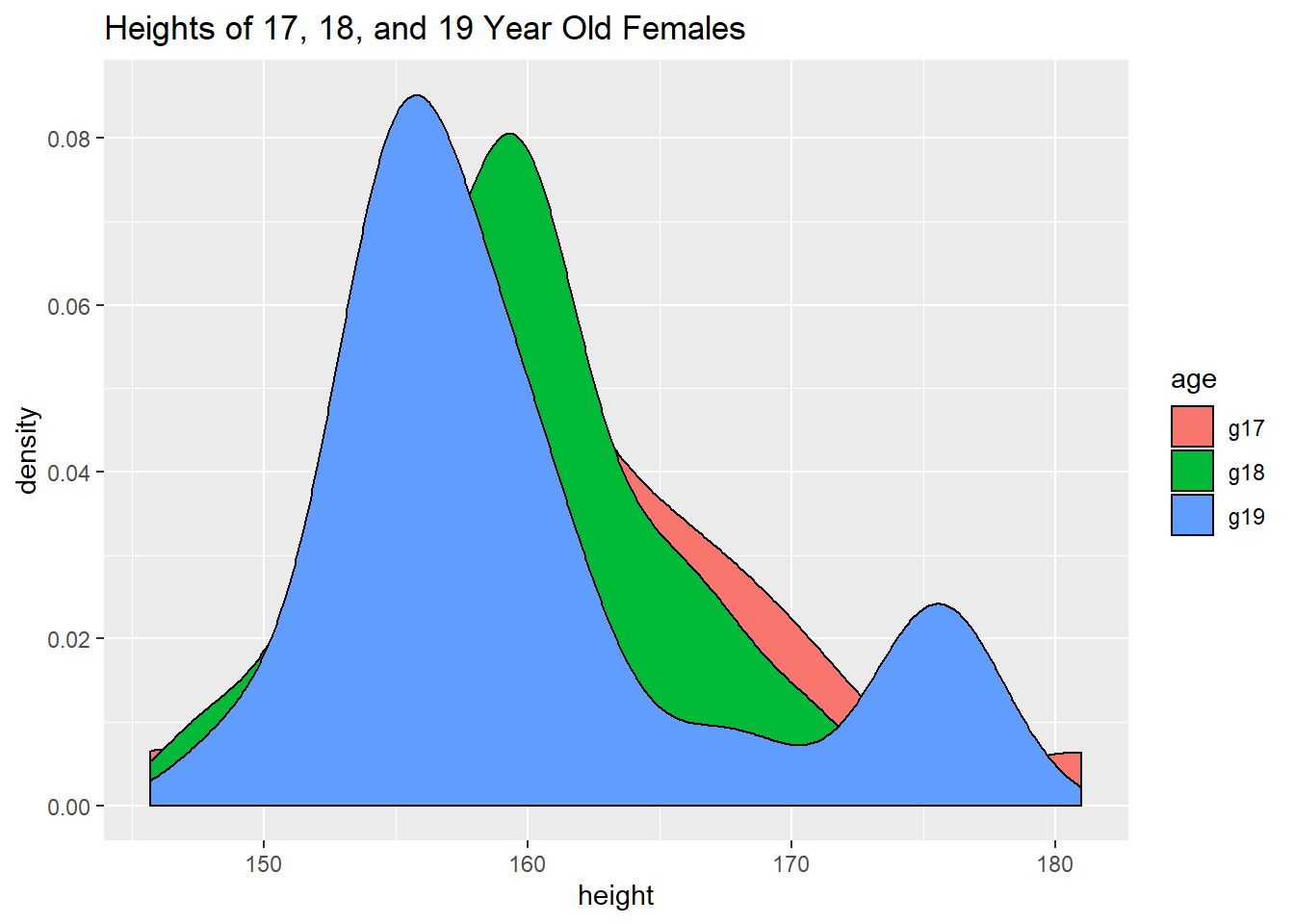## ## Call: ## lm(formula = height ~ age, data = df3) ## ## Residuals: ## Min 1Q Median 3Q Max ## -15.533 -4.532 -1.378 2.722 19.770 ## ## Coefficients: ## Estimate Std. Error t value Pr(>|t|) ## (Intercept) 161.242 1.564 103.066 <2e-16 *** ## ageg18 -2.127 2.212 -0.961 0.341 ## ageg19 -1.417 2.212 -0.640 0.525 ## --- ## Signif. codes: 0 '***' 0.001 '**' 0.01 '*' 0.05 '.' 0.1 ' ' 1 ## ## Residual standard error: 6.996 on 57 degrees of freedom ## Multiple R-squared: 0.01653, Adjusted R-squared: -0.01798 ## F-statistic: 0.479 on 2 and 57 DF, p-value: 0.6219 This linear model uses the 17 year olds as the reference category, and is: $y = 161.242 - (2.127 \:*\: {ageg18}) - (1.417 \:*\: {ageg19})$ • If we want to predict the height of the 17 year olds, ageg18 and ageg19 are both going to be zero as a 17 year old is not in either of these groups. The linear model reduces to y=161.242, which says that a good estimate for the height of those people is the mean of our sample of n=20 people who are age 17. • If we want to predict the height of the 18 year olds, ageg18 equals 1 but ageg17 and ageg19 are zero. The model reduces to y=161.242-2.127, which predicts the height of 18 year olds to be 159.115 cm, also the group mean. • If we want to predict the height of the 19 year olds, ageg19 equals 1 but ageg17 and ageg18 are zero. The model reduces to y=161.242-1.417 or around 160 cm, the group mean. ### One-Way ANOVA The ANOVA and Tukey HSD should also show no difference between the groups. This means a large p-value (greater than 0.05, but preferably much much greater) and all confidence intervals crossing the vertical dashed zero line in the Tukey plot: ## Df Sum Sq Mean Sq F value Pr(>F) ## age 2 46.9 23.45 0.479 0.622 ## Residuals 57 2790.2 48.95 ## Tukey multiple comparisons of means ## 95% family-wise confidence level ## ## Fit: aov(formula = height ~ age, data = df3) ## ##$age
##               diff       lwr      upr     p adj
## g18-g17 -2.1266601 -7.450780 3.197460 0.6041619
## g19-g17 -1.4167479 -6.740868 3.907372 0.7985327
## g19-g18  0.7099123 -4.614207 6.034032 0.9448832